Test: Division Algorithm for Polynomials

# Test: Division Algorithm for Polynomials - Class 10

Test Description

## 20 Questions MCQ Test Mathematics (Maths) Class 10 - Test: Division Algorithm for Polynomials

Test: Division Algorithm for Polynomials for Class 10 2023 is part of Mathematics (Maths) Class 10 preparation. The Test: Division Algorithm for Polynomials questions and answers have been prepared according to the Class 10 exam syllabus.The Test: Division Algorithm for Polynomials MCQs are made for Class 10 2023 Exam. Find important definitions, questions, notes, meanings, examples, exercises, MCQs and online tests for Test: Division Algorithm for Polynomials below.
Solutions of Test: Division Algorithm for Polynomials questions in English are available as part of our Mathematics (Maths) Class 10 for Class 10 & Test: Division Algorithm for Polynomials solutions in Hindi for Mathematics (Maths) Class 10 course. Download more important topics, notes, lectures and mock test series for Class 10 Exam by signing up for free. Attempt Test: Division Algorithm for Polynomials | 20 questions in 20 minutes | Mock test for Class 10 preparation | Free important questions MCQ to study Mathematics (Maths) Class 10 for Class 10 Exam | Download free PDF with solutions
 1 Crore+ students have signed up on EduRev. Have you?
Test: Division Algorithm for Polynomials - Question 1

### The expression that should be subtracted from the polynomial f(x) = x4 + 2x3-13x2 – 12x + 21 so that the resulting polynomial is exactly divisible by g(x) = x2 – 4x + 3 is​

Detailed Solution for Test: Division Algorithm for Polynomials - Question 1

Step-by-step explanation:

Given: Dividend =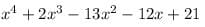Divisor =  x2 - 4x + 3

Solution :

Since we know that :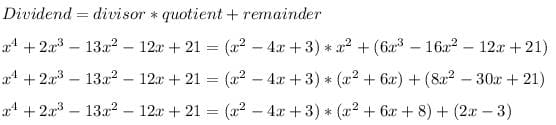Since the remainder is  2x-3

So, 2x-3 must be subtracted from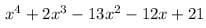so that the resulting  polynomial is exactly divisible by x2 - 4x + 3

Test: Division Algorithm for Polynomials - Question 2

### If (x + 1) is a factor of x2 – 3ax + 3a – 7, then the value of a is

Detailed Solution for Test: Division Algorithm for Polynomials - Question 2

Since (X+1) is a factor of x2 - 3ax + 3a - 7

Therefore , x+1=0

or, x=-1

Putting the value of x in the the p(x) = x2 - 3ax + 3a - 7

or, (-1)2 -3(a)(-1)+3a-7=0

1+3a+3a-7=0

6a=6

or, a=1

Therefore the  value of a=1.

Test: Division Algorithm for Polynomials - Question 3

### The value of quadratic polynomial f (x) = 2x2– 3x- 2 at x = -2 is ……​

Detailed Solution for Test: Division Algorithm for Polynomials - Question 3

We put the value x=-2 in the polynomial 2x2-3x-2
f(-2)=2(-2)2-3(-2)-2=2*4+6-2=12

Test: Division Algorithm for Polynomials - Question 4

If the degree of the dividend is 5 and the degree of the divisor is 3, then the degree of the quotient will be

Detailed Solution for Test: Division Algorithm for Polynomials - Question 4

The degree of the divisor is 2

Step-by-step explanation:

x5÷x3=x2

Test: Division Algorithm for Polynomials - Question 5

The value of 155 mod 9 is

Detailed Solution for Test: Division Algorithm for Polynomials - Question 5

By the Division algorithm 155 = 9(17) + 2. Where remainder is 155 mod 9.

Test: Division Algorithm for Polynomials - Question 6

On dividing f(x) = x3 – 3x2 + x + 2 by a polynomial g(x) the quotient and remainder q(x) and r(x) are (x – 2) and (-2x + 4) respectively, then g(x) is​

Detailed Solution for Test: Division Algorithm for Polynomials - Question 6

Divisor*Quotient+Remainder= Divident
g(x)(x-2)+(-2x+4)=x3-3x2+x+2
g(x)(x-2)=x3-3x2+x+2+2x-4
g(x)(x-2)=x3-3x2+3x-2
g(x)=x3-3x2+3x-2/(x-2)
g(x)=x2-x+1

Test: Division Algorithm for Polynomials - Question 7

The three zeroes of the polynomial 2x3 + 5x2 – 28x – 15 _____

Detailed Solution for Test: Division Algorithm for Polynomials - Question 7

Since the solution is not a complex number, it is a real number. Since rational numbers include all integers, natural numbers and fractions , So the numbers are rational numbers.

Test: Division Algorithm for Polynomials - Question 8

If two of the zeroes of the polynomial f (x) = x4 – 3x3 – x2 + 9x – 6 are -√3 and √3 then all the zeroes are​

Test: Division Algorithm for Polynomials - Question 9

If (x + 1) is a factor of 2x3 + ax2 + 2bx + 1, then find the values of a and b given that 2a – 3b = 4.​

Detailed Solution for Test: Division Algorithm for Polynomials - Question 9

Given is the value of a and b.

given that (x+1) is a factor of p(x)

therefore, -1 is a zero of given p(x)

p(x) = 2x3 +ax2 +2bx +1

substituting the value of -1 in the given p(x), we get

p(x)=2*(-1)3 +a *(-1)2 +2*b*(-1)+ 1

= -2 + a -2b + 1

= -1 + a - 2b

or,a - 2b = 1

also given that 2a - 3b = 4

so we got two equations;

a - 2b = 7 ...(1)

2a - 3b = 4 ...(2)
(1)* (2)* (4)- 2 = 2a - 4b = (3)

(2)* 1 = 2a - 3b = 4 (4)

(4) -  (3) = [2a - 2a ] + [-3b - (-4b)] = 4 - 2

-3b + 4b = 2

therefore b = 2

substituting the value of bin (3)

2a - 4b = 2

2a - (4*2) = 2

2a - 8 = 2

2a = 2 + 8

2 = 10

a = 10 / 2

therefore a = 5

so we get the value of a and b

that is ; a = 5 and b = 2

Test: Division Algorithm for Polynomials - Question 10

Which of the given is the set of zeroes of the polynomial p(x) = 2x3 + x2 – 5x + 2

Detailed Solution for Test: Division Algorithm for Polynomials - Question 10

We have the polynomial 2x3+x2-5x+2
By Hit and trial method, x=1 is the solution.
Dividing the polynomial by x-1
We get quotient as = 2x2+3x-2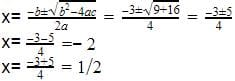Test: Division Algorithm for Polynomials - Question 11

The value of p when x3 + 9x2 + px – 10 is exactly divisible by (x+ 2) is ____

Test: Division Algorithm for Polynomials - Question 12

When the polynomial f(x) = 4x3 + 8x2 + 8x + 7 is divided by the polynomial g(x) = 2x2 – x + 1, the quotient and the remainder are​

Test: Division Algorithm for Polynomials - Question 13

When x2 – 2x + k divides the polynomial x4 – 6x3 + 16x2 – 25x + 10, the remainder is (x + a). The value of a is _________

Detailed Solution for Test: Division Algorithm for Polynomials - Question 13

Given that the remainder is (x + a)
⇒ (4k – 25 + 16 – 2k)x + [10 – k(8 – k) ] = x + a
⇒ (2k – 9)x + [10 – 8k + k2 ] = x + a
On comparing both the sides, we get
2k  – 9 = 1
⇒ 2k = 10
∴ k = 5
Also 10 – 8k + k2 = a
⇒ 10 – 8(5) + 52 = a
⇒ 10 – 40 + 25 = a
∴ a = – 5

Test: Division Algorithm for Polynomials - Question 14

If the degree of the divisor g(x) is one then the degree of the remainder r(x) is

Detailed Solution for Test: Division Algorithm for Polynomials - Question 14

Degree of remainder is one less than the degree of the divisor because if the remainder is of the same degree then it can be divided further , its just like when the divisor is a number the remainder is less than the number because if it is more than or equal to that number it can further be divided. So, the degree is zero.

Test: Division Algorithm for Polynomials - Question 15

If the polynomial (2x + 3) is a factor of the polynomial 2x3 + 9x2 – x – b. The value of b is_______

Detailed Solution for Test: Division Algorithm for Polynomials - Question 15

let the given polynomial be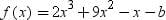since 2x+3=0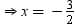if (2x+3) is a factor of f(x). therefore by the factor theorem f(-3/2) =0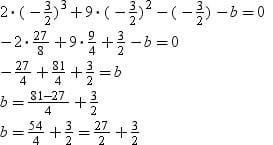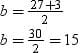Test: Division Algorithm for Polynomials - Question 16

If two of the zeros of the polynomial x4 – 17x3 + 90x2 – 166x + 92 are (7 + √3) and (7 – √3), then the other two zeros are _________​

Test: Division Algorithm for Polynomials - Question 17

If f(x) is divided by g(x), g(x) ≠ 0, then there exist two polynomials q(x) and r(x) such that​

Detailed Solution for Test: Division Algorithm for Polynomials - Question 17

Dividend = Divisor × Quotient + Remainder.
When we divide f(x) by g(x) then q(x) is quotient and r(x) is remainder.
Therefore, f(x) = g(x) × q(x) + r(x).

Test: Division Algorithm for Polynomials - Question 18

The expression that should be added to the polynomial f(x) = x4 + 2x3 – 2x2 + x + 1, so that it should be exactly divisible by (x2 + 2x – 3) is​

Test: Division Algorithm for Polynomials - Question 19

When x3 – 3x2 + 5x – 3 is divided by x2 – k , the remainder is 7x + a . Then the value of k is_____

Test: Division Algorithm for Polynomials - Question 20

If two zeroes of a polynomial 4x4 -20x3 +23 x2 + 5x – 6 are ½ and – ½, the how many more zeroes does it have?

Detailed Solution for Test: Division Algorithm for Polynomials - Question 20

The polynomial with degree 4 is a biquadratic equation. And a polynomial has no. of zeros equal to the degree of the polynomial. So total no. of zeros are 4. 2 zeros are already provided to us , so it has 2 more zeros.

## Mathematics (Maths) Class 10

115 videos|478 docs|129 tests
Information about Test: Division Algorithm for Polynomials Page
In this test you can find the Exam questions for Test: Division Algorithm for Polynomials solved & explained in the simplest way possible. Besides giving Questions and answers for Test: Division Algorithm for Polynomials, EduRev gives you an ample number of Online tests for practice

## Mathematics (Maths) Class 10

115 videos|478 docs|129 tests